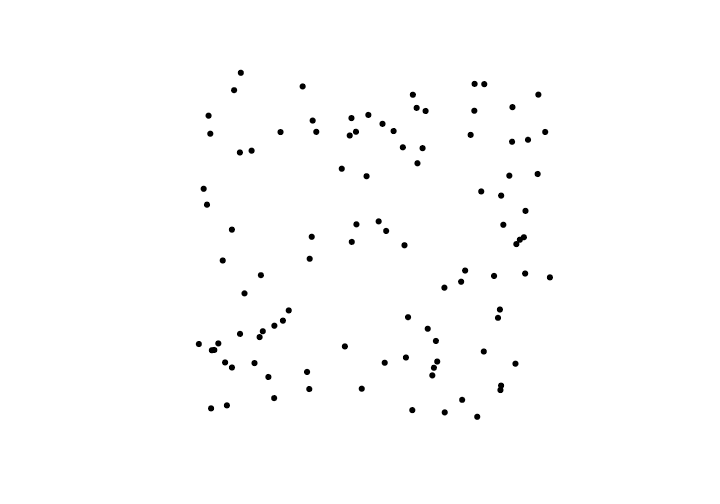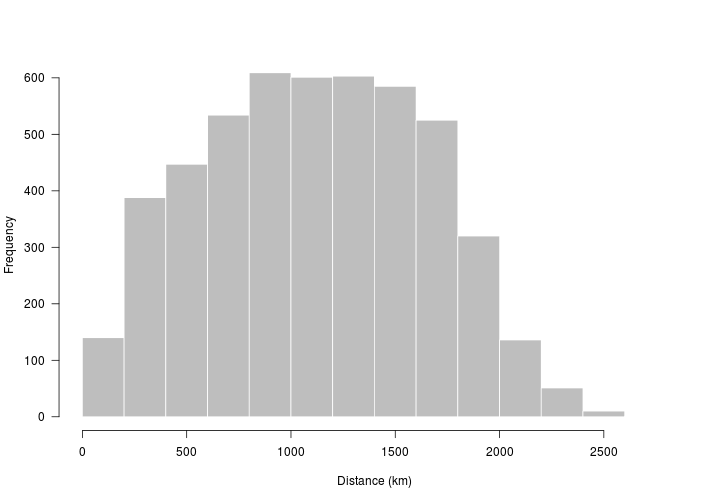# Introduction to spatial points in R

This activity will introduce you to working with spatial points in R.

R Skill Level: Intermediate - this activity assumes you have a working knowledge of `R`

# Objectives & Goals

Upon completion of this activity, you will:
• know how to create and write spatial points
• Be able to project spatial points
• Be able to calculate distances between points

### Required packages

To complete the following activity you will need the following packages installed: raster sp rgeos

#### Installing the packages

If installing these packages for the first time consider adding `dependencies=TRUE`
• `install.packages("raster")`
• `install.packages("sp")`
• `install.packages("rgeos")`

• Last modified: 2019-09-20 18:26:28

# What are spatial points?

Spatial points are a set of spatially explicit coordinates that represent a geographic location. Each point represents a location on a surface. Spatial points are created from a series of x and y coordinates. Spatial points can be combined with data frames to create what’s called a `SpatialPointsDataFrame`. The difference between `SpatialPoints` and `SpatialPointsDataFrame` are the attributes that are associated with the points. `SpatialPointsDataFrames` have additional information associated with the points (e.g., site, year, individual, etc.) while `SpatialPoints` contain only the spatial information about the point.

# Creating & writing spatial points

## Spatial Points in R

Let’s begin by creating a set spatial points layer from scratch. We’ll use the `sp` package to make a `SpatialPoints` object using randomly generated XY coordinates. Once we create a `SpatialPoints` object in R - we’ll take a closer look at its metadata and structure.

load the `sp` package if you haven’t already done so. If you need to install the `sp` package - see how to do that here

``````# load library
library(sp)
``````

Now that the `sp` library is loaded we can use the `SpatialPoints()` function to create a `SpatialPoints` object in `R`.

``````# Generate 100 random X and Y coordinates
# with longitude and latitude in the familiar
# degrees

x_coords <- runif(n = 100, min = -100, max = -80)
y_coords <- runif(n = 100, min = 25, max = 45)

# Have a look at the first coordinates
head(cbind(x_coords,y_coords))
``````
``````##       x_coords y_coords
## [1,] -94.08413 43.83492
## [2,] -84.31071 42.45115
## [3,] -82.96326 30.66174
## [4,] -80.70545 38.85120
## [5,] -97.65633 40.07562
## [6,] -82.78829 26.80323
``````

Now that we have generated random coordinates we can make those data spatially explicit. We’ll use the `SpatialPoints` function in the `sp` package to do that. Before we use the function let’s see what arguments we need to pass to `SpatialPoints`.

``````args("SpatialPoints")
``````
``````## function (coords, proj4string = CRS(as.character(NA)), bbox = NULL)
## NULL
``````

The `SpatialPoints` function is looking for coordinates (`coords`), a projection / datum argument (`proj4string`) and a bounding box (`bbox`). Both `proj4string` and `bbox` have preset values so we don’t need to specify them - it’ll use the defaults. Let’s use those options for now.

The `coords` input looking for a specific type of input. It needs a matrix or data.frame where the first column is longitude and second is latitude. Note - the order is LONGITUDE then LATITUDE.

``````# coords = c(longitude,latitude)

firstPoints <- SpatialPoints(coords = cbind(x_coords,y_coords))
``````

Let’s have a look at what we just created.

``````str(firstPoints)
``````
``````## Formal class 'SpatialPoints' [package "sp"] with 3 slots
##   ..@ coords     : num [1:100, 1:2] -94.1 -84.3 -83 -80.7 -97.7 ...
##   .. ..- attr(*, "dimnames")=List of 2
##   .. .. ..\$ : NULL
##   .. .. ..\$ : chr [1:2] "x_coords" "y_coords"
##   ..@ bbox       : num [1:2, 1:2] -100 25 -80 44.6
##   .. ..- attr(*, "dimnames")=List of 2
##   .. .. ..\$ : chr [1:2] "x_coords" "y_coords"
##   .. .. ..\$ : chr [1:2] "min" "max"
##   ..@ proj4string:Formal class 'CRS' [package "sp"] with 1 slot
##   .. .. ..@ projargs: chr NA
``````

We can plot the points in space by simply using the `plot` function.

``````plot(firstPoints, pch = 19)
``````Back to top

## Writing a shapefile

We can save our `firstPoints` object as a shapefile using the `raster` package. The `shapefile` function in the `raster` package is very convienent in that it can both read a shapefile into `R` but it can also write a `SpatialPoints` or other spatial object classes (lines, polygons, etc.) to a shapefile.

``````library(raster)
shapefile(x = firstPoints, file = "path/to/output/file.shp")
``````

Back to top

## Calculate distance between points

One task that may be useful now that we have spatial points is calculating the distance between points either within the same layer or between two layers. Since we already have a SpatialPoints file, we’ll calculate the distance between points. We need to be a little careful here. First, we need to make sure we’re calculating the distance we intend to calculate. Let’s start simple and get Euclidean distances in the units of the SpatialPoints layer. If in meters, it’ll return meters. If degrees, it’ll return degrees.

``````# longlat = FALSE returns Euclidean distance
euclidDist <- sp::spDists(firstPoints,longlat = FALSE)
``````
``````##  num [1:100, 1:100] 0 9.87 17.24 14.28 5.19 ...
``````

Another option is to calculate the GreatCircle distance.

``````# longlat = TRUE returns GreatCircle distance
gcDist <- sp::spDists(firstPoints,longlat = TRUE)
``````
``````##  num [1:100, 1:100] 0 809 1760 1247 512 ...
``````We’ll dive a bit deeper into point-based analyses in spatial predicates and in the activities